Home > CC2 > Chapter 9 > Lesson 9.1.2 > Problem9-31

9-31.
1. The circle shown at right has a diameter of 20 cm. Homework Help ✎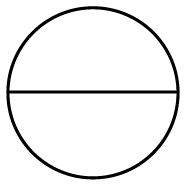1. What measurement do you need to find in order to calculate the area inside the circle?

2. Find the area inside the circle using the formula A = r2π. Write your answer as a product of r2 and π, and as an approximation using π = 3.14.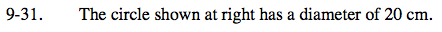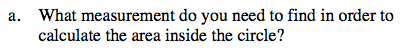Recall the equation for area from the Math Notes box in this lesson.

A = r2 π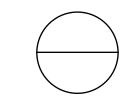What measurement is missing in this equation?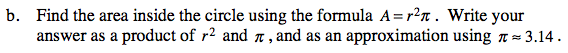Diameter = 2 (radius)

Solve for r.

Substitute the newly calculated radius into the area formula.

20 = 2 r

A = (10)2 π

314 cm2